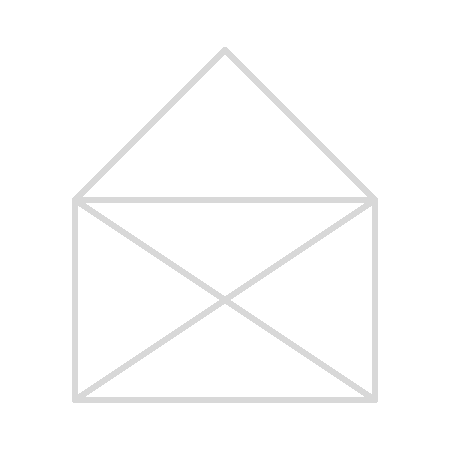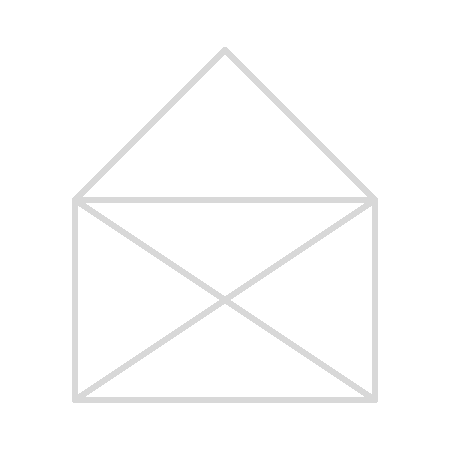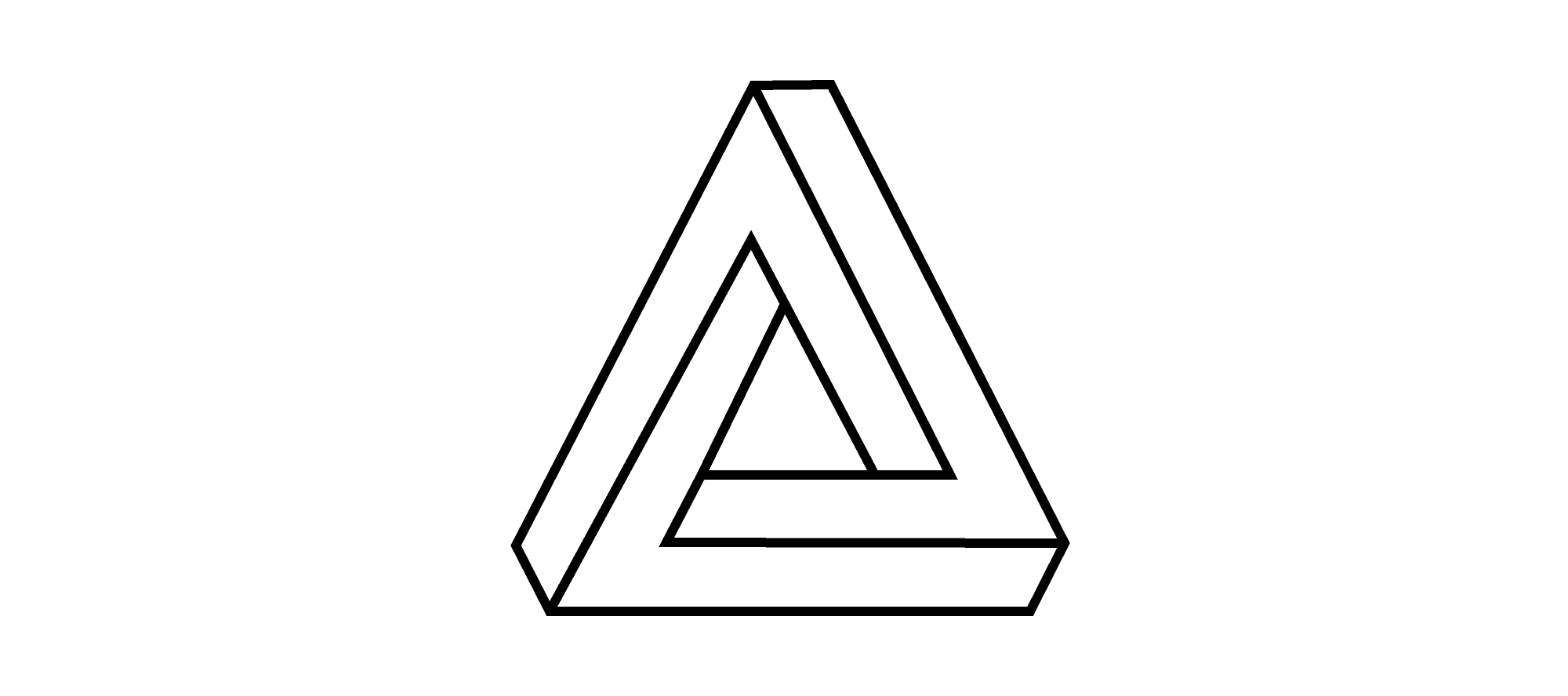Back

## Trace the Impossible Triangle

Let's say we wanted to trace the shape below, but under these rules:

1. You can start anywhere on the figure.
2. You cannot lift your writing tool once you start tracing.
3. You cannot trace over any segment more than once.Is it possible? Keep reading to find out, or just jump ahead to today's challenge if you feel up to a similar but trickier problem.

Let's look at a few approaches.We didn't find a solution here, but our failed attempts didn't prove that a solution is impossible. There are hundreds of possible ways we could try to trace the shape, so blind guessing doesn't seem like the best approach here. When solving a problem, it's sometimes helpful to focus on one piece rather than the whole thing. Let's think about what happens at the vertices of the shape as we trace it.

When we come to a vertex as we're tracing the path, we have to enter on one line and then leave on another, unless we are starting or stopping at that vertex. In other words, each path through a vertex needs two different lines. So, for any vertex with two, four, six, etc. lines, for every way in, there's always a way out. What if a vertex has an odd number of lines passing through it? This means there's a way into the vertex for which there's no way out, or vice versa.

When we start at a vertex, we leave without entering first, which lets us add a single line to that vertex. So, the vertex at which we begin could have an odd number of connections. Similarly, when we finish our path, we draw the line to a vertex and do not need to leave, so the final vertex could also have an odd number of connections.

In this problem, only two of the vertices in the image have an odd number of connections — the bottom corners. So, any path completely tracing the shape must start at one of the bottom corners and end at the other.Try applying this insight to the problem below.

# Today's ChallengeUsing the rules below, you'd like to be able to trace the figure above.

• You can start at any intersection point on the figure.
• You cannot lift your writing tool once you start tracing.
• You cannot trace over any segment more than once.

It might be impossible to trace the figure in this way. What is the least number of straight segments that you would have to leave untraced?×